• Among algorithms, coming up with cost functon, with optimal objective.
• So we want to use the gradient descent, one of the algorithm that will minimize cost function.
• Now, gradient descent, will become computationally expensive as we increase the data.
• So we will alter the algorithm into something called Stochastic Gradient Descent
• Come up solution to deal larger datasets,.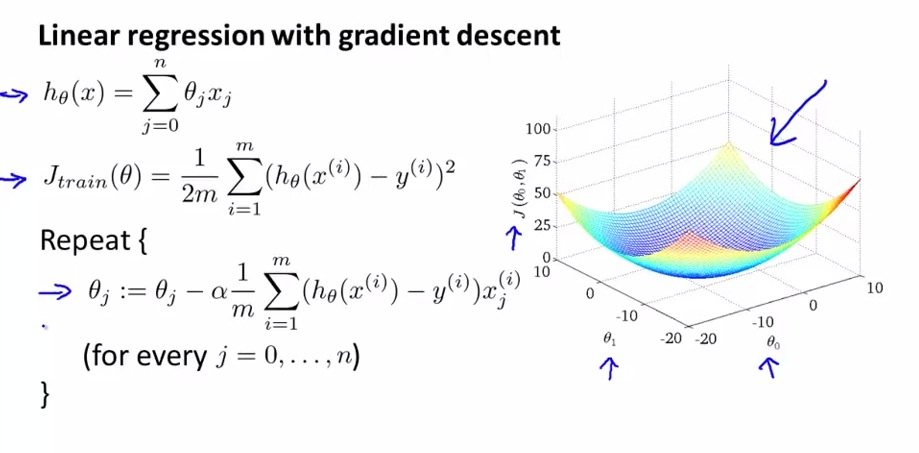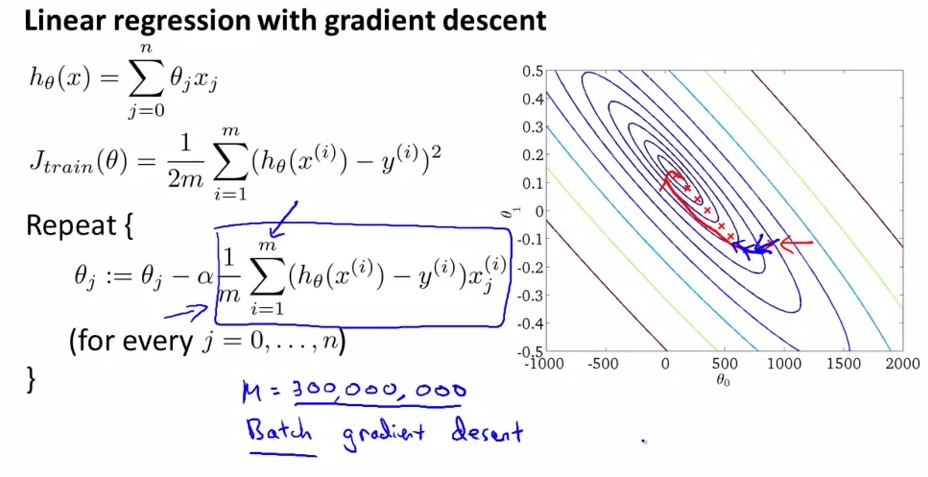• recap of gradient desect and the cost function
• In this video, we will stick with linear regression, although this concept of gradient descent can be easily applicable in many other algorithms with logistic regression, neural networks, etc.

• Take projection to global minimum
• computing derrivative term is expensive becosse of iterating to hundreds millions of data
• particular GD - Batch gradient descent
• called batch = look up all training example
• What earlier we do is looping into hundreds of millions, because the data much larger data not full enough emory.
• take a long time for algoirth m to converge, sum 300 hundred millions every iteration.
• come up with solution for long iteration, we want to step only 1 training example on each iteration.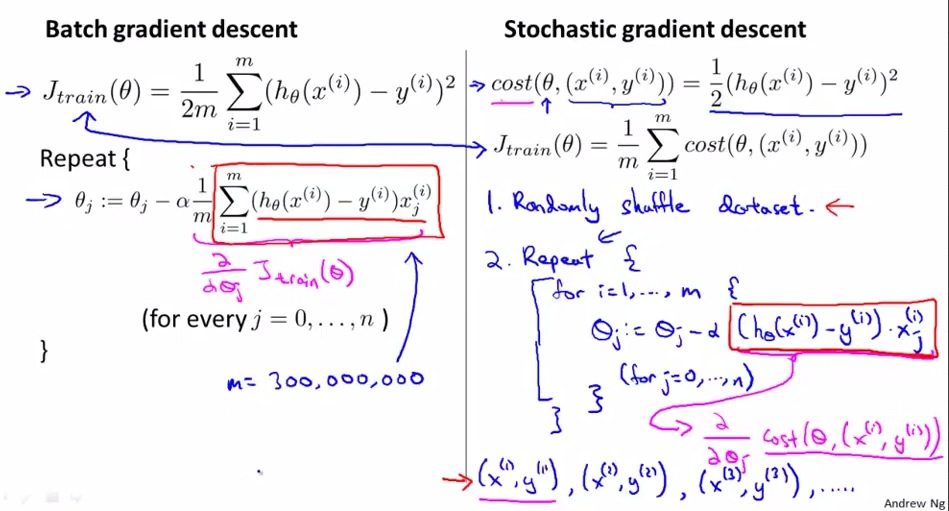• Now,here’s the difference between batch and stochastic
• in batch, we try to take all examples in one iteration, making it have to wait m training examples(e.g. m = 300.000.000) just to produce gradient descent(partial derivative) and minimize the thetas.
• Imagine we have to wait 300.000.000 just to change a bit of cost function, just once.
• In Stochastic, we are simply using 1 training example.
• So gradient descent just take one training example each iteration, making it utterly fast!
• Moreover, we modify the cost function in an instant, because we don’t have to wait summation over all data examples.
• In batch 1 iteration with all data examples, with stochastic m iteration with one data example. This way stochastic can progress quickly(show the progress to global minimum in ms time) instead of waiting like batch gradient descent.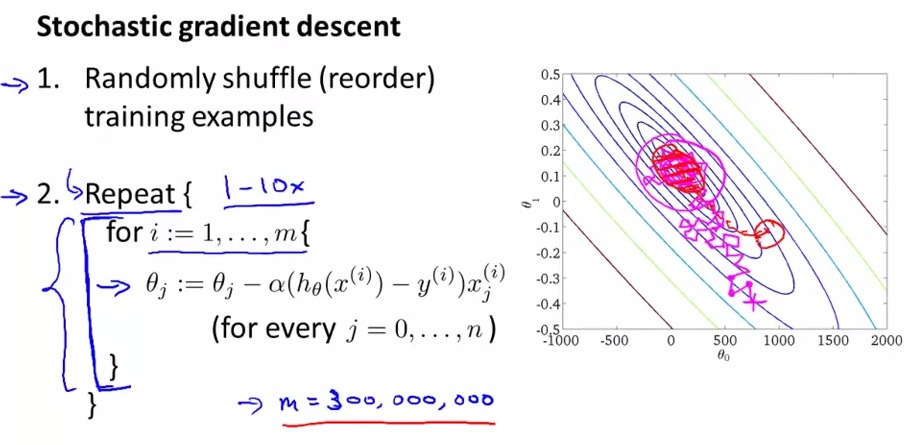• The red shown on the graph is produced by batch gradient descent. We can see it that taking all example each iteration making baby step towards global minimum, but always converge to global minimum
• In the contrary, the stochastic with each iteration, because each example is different, we may end up as shown in magenta.
• Stochastic will taking the baby step, but with stochastic way to global minimum. No taking definite path like batch GD.
• Stochastic will not always converge to global minimum, and can be circling around the global minimum, but it should be fine. It still produce perfectly fine global minimum, as shown in circle magenta at global minimum.
• And how many repeat(outer loop) we should get. At 300.000.000 example, 1 repeat could be fine. But in general 1-10x repeat.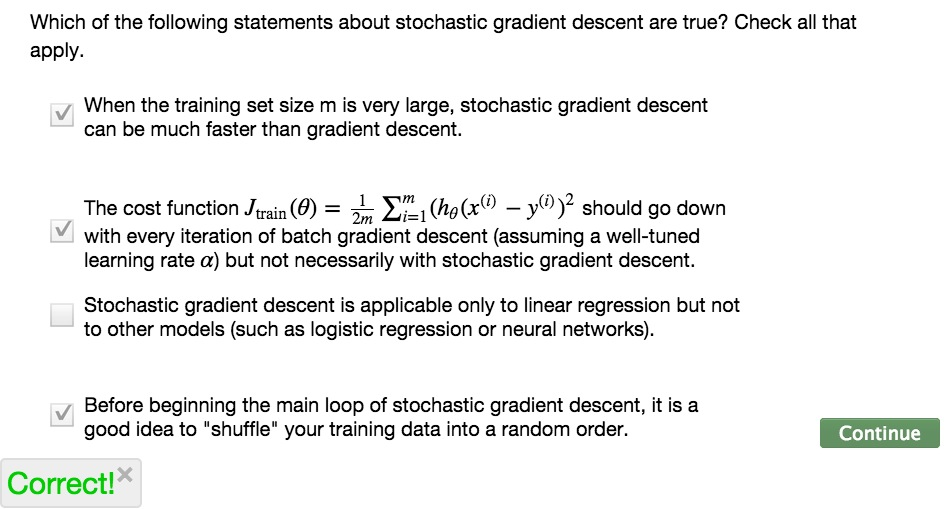• Hopefully when we implemented like this, we will be able to cover huge data example with faster performance